# Constructing Angles with a Protractor

Constructing angles is an important part of geometry as this knowledge is extended for the construction of other geometric figures as well, primarily the triangles. Before talking about constructing angles with a protractor, let us quickly recall angles and their types. An angle is made when two rays start from a common point. This point is called the vertex of the angle and the two rays forming the angle are called its arms or sides. Depending on the inclination between its two arms, an angle may be acute (less than 90 degrees), obtuse (more than 90 degrees) or right-angled (exactly 90 degrees).

• constructing angles of given measure as well as of unknown measure using geometric tools such as the
• Protractor
• Compasses
• Ruler
• How to construct a 75-degree angle

Also check: Examples of Construction of Different Angles

## The Protractor

It is a semi-circular disc used to draw and measure angles. It is graduated from 0 to 180 degrees and can be directly used to measure any angle within the range. It has two sets of markings, 0 to 180 degrees from left to right and vice versa.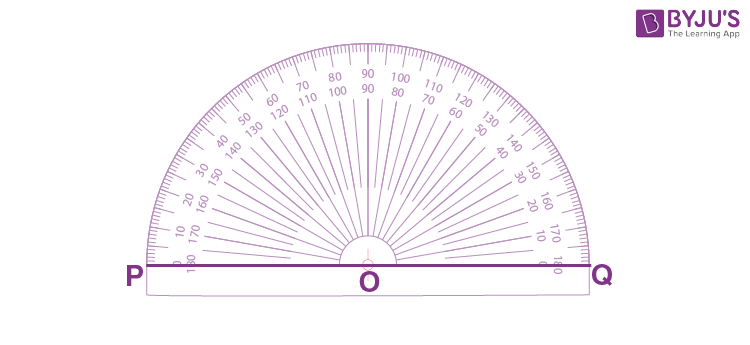## Constructing Angles Using a Protractor

For constructing angles of any given measure, be it an acute, an obtuse or a right-angle, the simplest method is by using a protractor. Let us say, you are asked to construct an angle of 120 degrees. The required steps are:

Step 1: Draw a line segment BC, which is one of the arms of the angle that is to be constructed.Step 2: Place the protractor with its point O on point B of the line segment BC.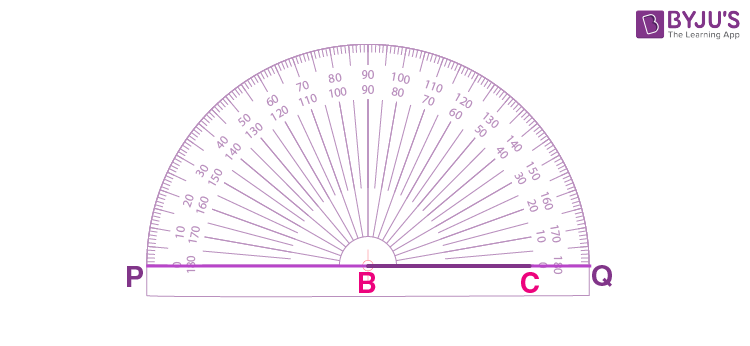Step 3: Align OQ along the edge BC.

Step 4: The protractor has two-way markings. We consider the scale which has 0 degrees near point C for construction. Mark point A next to the 120 degrees mark on the scale.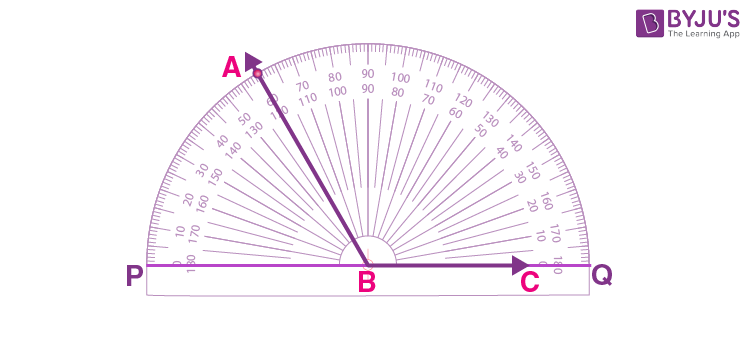Step 5: Join points A and B. ∠ABC = 120 degrees is the required angle.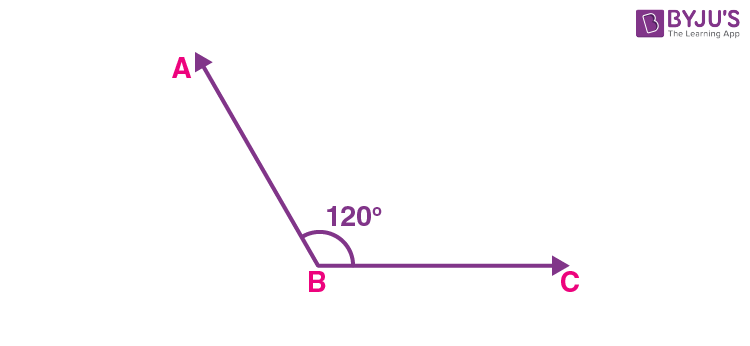## Constructing Angles Using Compass

Constructing angles of unknown measure is basically copying a given angle whose measure is not known. We accomplish this task using compasses. Let us say that you are given ∠BAC that you are supposed to copy.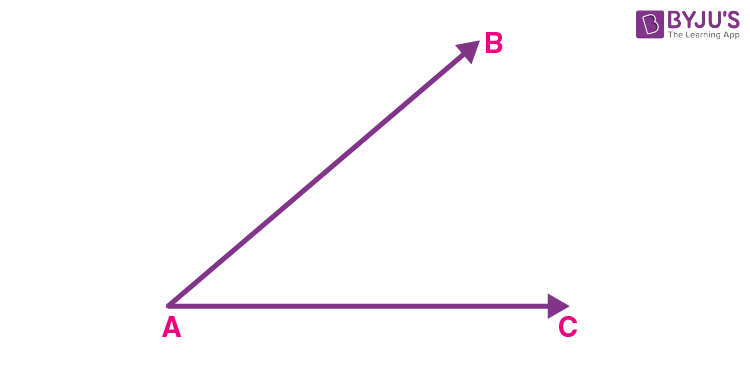The steps to construct angles using compass are given below:

Step 1: Draw a line PQ. Point P is the vertex of the copied angle.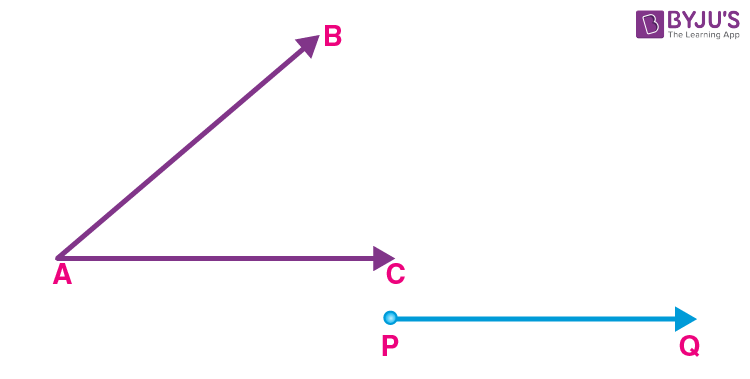Step 2: Place the compass pointer at point A and make an arc that cuts arms AC and AB at points K and J respectively.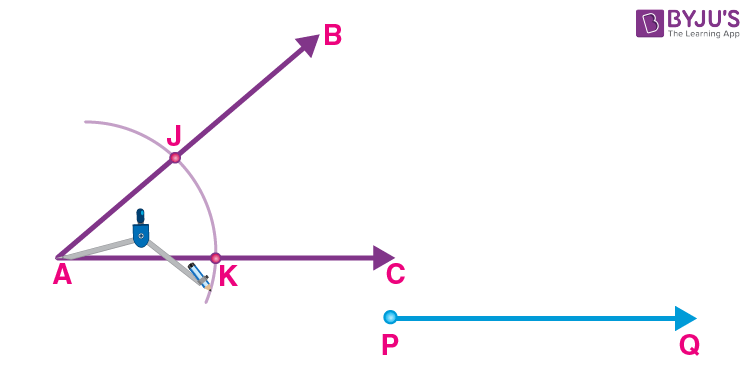Step 3: Without changing the radius of the compass, cut an arc on PQ at point M.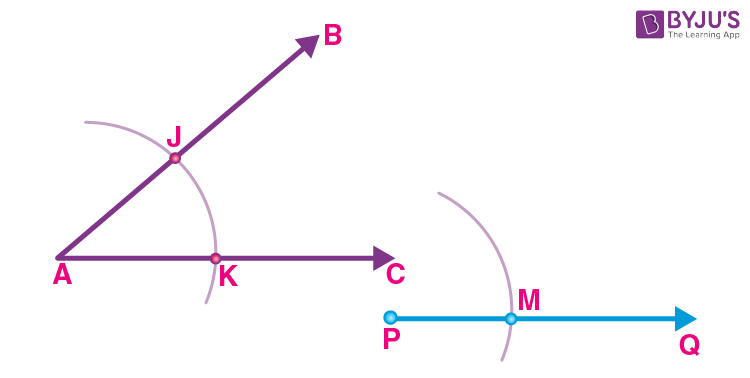Step 4: Adjust the compass such that the pointer is placed at K and the pencil head at J.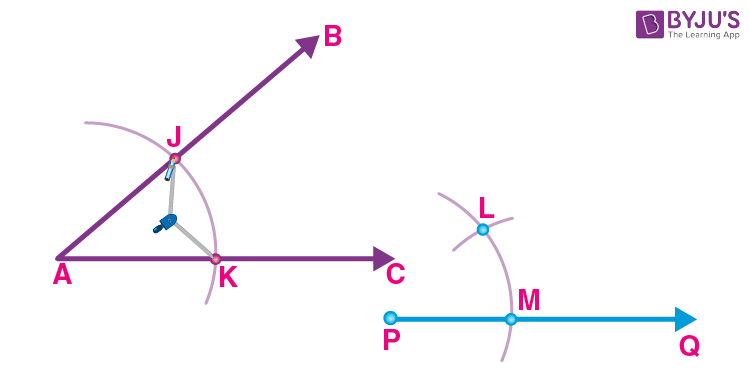Step 5: With the same radius, draw an arc on the first arc with the compass pointer at M. Mark the intersecting point as L.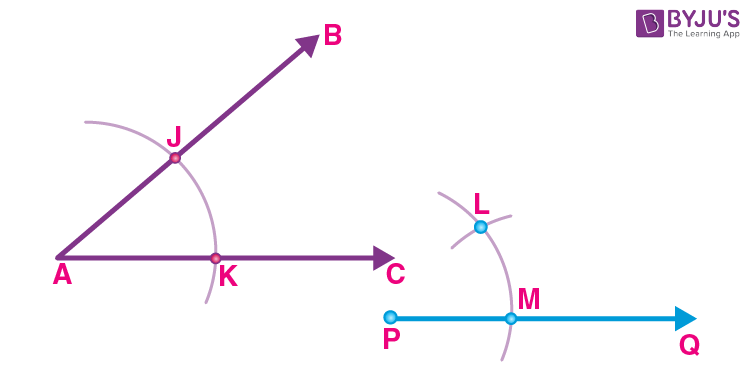Step 6: Join the points P and L using a ruler. Extend the line up to R.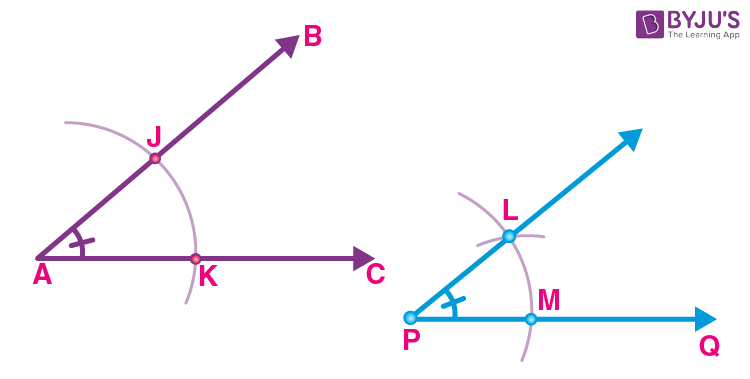Step 7: ∠RPQ is the required angle.

## Video Lesson on Constructing Angle

#### For More Information On Constructing An Angle Of 60 Degrees, Watch The Below Video:## How to Construct 75 degree Angle

To construct a 75-degree angle, we need to produce an angle bisector in between 90-degree angle. Let’s learn the steps to construct a 75-degree angle.

• Step 1: Draw a line segment with endpoint O and A.
• Step 2: Draw an arc with O as centre cutting the line segment OA at point B with a compass.
• Step 3: Keeping the radius same, Draw an arc with B as centre cutting the arc at C.
• Step 4: Keeping the radius same, and C as the center, draw an arc intersecting the arc drawn in the previous step at D.
• Step 5: With any radius, Draw two arcs with C and D as a center. Intersect these two arcs at E.
• Step 6: Join OE. angle EOA is the angle with measurement of 90 degrees.
• Step 7: Now the line OE intersect the arc at the point F.
• Step 8: Taking F and C as a center, make an arc with a radius of more than half of the measurement FC. The arc intersects at point H.
• Step 9: Join the point H and O. Angle HOA is the angle obtained of measurement 75 degrees.
• Step 10: Angle HOA is the desired angle.